# CuBIC - Cumulant Based Inference of higher-order Correlation¶

CuBIC is a statistical method for the detection of higher order of correlations in parallel spike trains based on the analysis of the cumulants of the population count. Given a list sts of SpikeTrains, the analysis comprises the following steps:

1. compute the population histogram (PSTH) with the desired bin size
>>> binsize = 5 * pq.ms
>>> pop_count = elephant.statistics.time_histogram(sts, binsize)

2. apply CuBIC to the population count
>>> alpha = 0.05  # significance level of the tests used
>>> xi, p_val, k = cubic(data, ximax=100, alpha=0.05, errorval=4.):

elephant.cubic.cubic(data, ximax=100, alpha=0.05)[source]

Performs the CuBIC analysis  on a population histogram, calculated from a population of spiking neurons.

The null hypothesis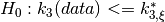is iteratively tested with increasing correlation order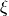(correspondent to variable xi) until it is possible to accept, with a significance level alpha, that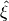(corresponding to variable xi_hat) is the minimum order of correlation necessary to explain the third cumulant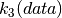.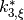is the maximized third cumulant, supposing a Compund Poisson Process (CPP) model for correlated spike trains (see ) with maximum order of correlation equal to.

Parameters: data : neo.AnalogSignal The population histogram (count of spikes per time bin) of the entire population of neurons. ximax : int The maximum number of iteration of the hypothesis test: if it is not possible to compute thebefore ximax iteration the CuBIC procedure is aborted. Default: 100 alpha : float The significance level of the hypothesis tests perfomed. Default: 0.05 xi_hat : int The minimum correlation order estimated by CuBIC, necessary to explain the value of the third cumulant calculated from the population. p : list The ordred list of all the p-values of the hypothesis tests that have been performed. If the maximum number of iteration ximax is reached the last p-value is set to -4 kappa : list The list of the first three cumulants of the data. test_aborted : bool Wheter the test was aborted because reached the maximum number of iteration ximax

References

Staude, Rotter, Gruen, (2009) J. Comp. Neurosci